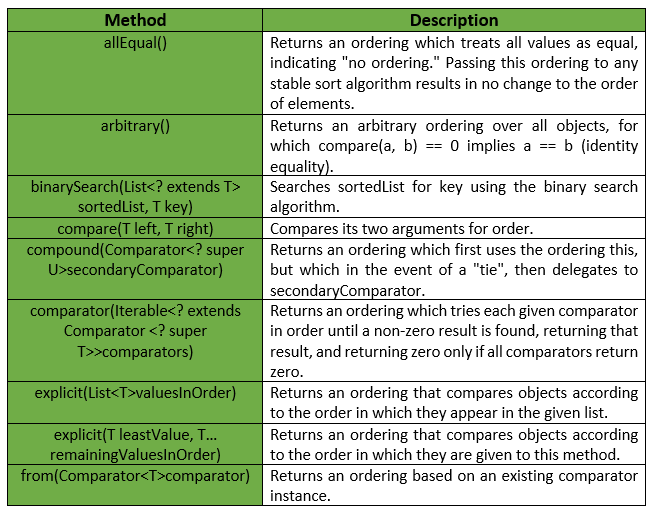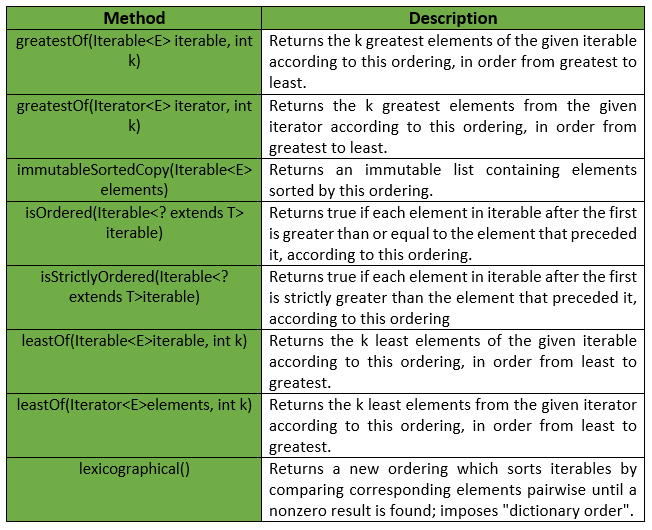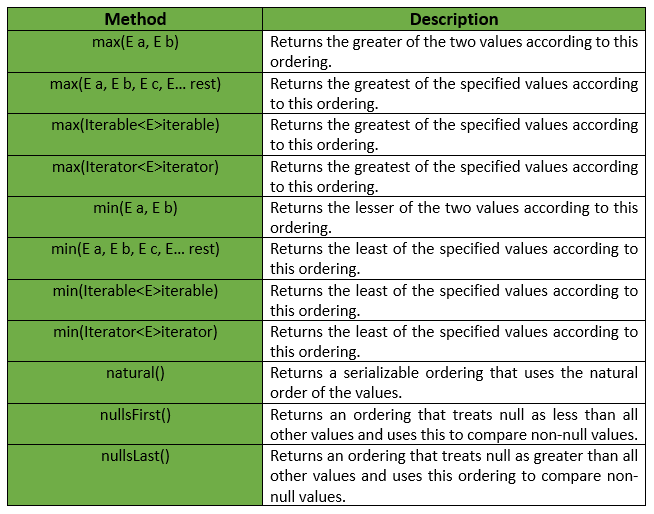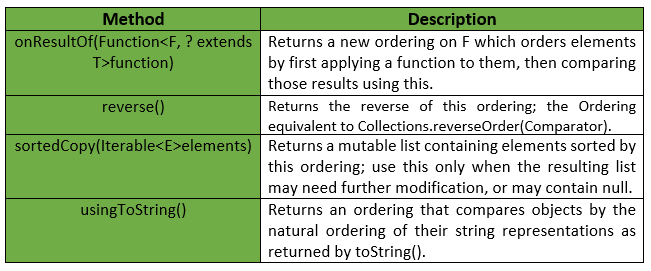# Ordering Class | Guava | Java

A comparator, with additional methods to support common operations. This is an “enriched” version of Comparator. The common ways to get an instance of Ordering are :

• Subclass it and implement compare(T, T) instead of implementing Comparator directly.
• Pass a pre-existing Comparator instance to from(Comparator).
• Use the natural ordering, natural().

Declaration : The declaration for com.google.common.collect.Ordering<T> class is :

```@GwtCompatible
public abstract class Ordering<T>
extends Object
implements Comparator<T>
```

Below given are some methods provided by Guava’s Ordering Class :Ordering() : This is a constructor of Ordering Class of Guava. It constructs a new instance of this class (only invokable by the subclass constructor, typically implicit). Some other methods provided by this Class are :Exceptions :

• explicit(List valuesInOrder) : NullPointerException if any of the provided values is null, IllegalArgumentException if valuesInOrder contains any duplicate values.
• explicit(T leastValue, T… remainingValuesInOrder) : NullPointerException if any of the provided values is null, IllegalArgumentException if any duplicate values.
• min(Iterator iterator) : NoSuchElementException if iterator is empty, ClassCastException if the parameters are not mutually comparable under this ordering.
• min(Iterable iterable) : NoSuchElementException if iterable is empty, ClassCastException if the parameters are not mutually comparable under this ordering.
• min(E a, E b) : ClassCastException if the parameters are not mutually comparable under this ordering.
• min(E a, E b, E c, E… rest) : ClassCastException if the parameters are not mutually comparable under this ordering.
• max(Iterator iterator) : NoSuchElementException if iterator is empty, ClassCastException if the parameters are not mutually comparable under this ordering.
• max(Iterable iterable) : NoSuchElementException if iterable is empty, ClassCastException if the parameters are not mutually comparable under this ordering.
• max(E a, E b) : ClassCastException if the parameters are not mutually comparable under this ordering.
• max(E a, E b, E c, E… rest) : ClassCastException if the parameters are not mutually comparable under this ordering.
• leastOf(Iterable iterable, int k): IllegalArgumentException if k is negative.
• leastOf(Iterator elements, int k) : IllegalArgumentException if k is negative.
• greatestOf(Iterable iterable, int k): IllegalArgumentException if k is negative.
• greatestOf(Iterator elements, int k) : IllegalArgumentException if k is negative.
• immutableSortedCopy : NullPointerException if any of elements (or elements itself) is null.

Some other methods provided by this class are :Example 1 :

 `// Java code to show implementation of ` `// Ordering class ` `import` `java.util.*; ` ` `  `import` `com.google.common.collect.Ordering; ` ` `  `class` `GFG { ` ` `  `    ``// Driver code ` `    ``public` `static` `void` `main(String args[]) ` `    ``{ ` ` `  `        ``// Creating a list of Integers ` `        ``List myList = ``new` `ArrayList(); ` ` `  `        ``myList.add(``new` `Integer(``12``)); ` `        ``myList.add(``new` `Integer(``3``)); ` `        ``myList.add(``new` `Integer(``78``)); ` `        ``myList.add(``new` `Integer(``50``)); ` `        ``myList.add(``new` `Integer(``6``)); ` `        ``myList.add(``new` `Integer(``70``)); ` `        ``myList.add(``new` `Integer(``18``)); ` `        ``myList.add(``new` `Integer(``9``)); ` `        ``myList.add(``new` `Integer(``10``)); ` ` `  `        ``// Displaying natural order of numbers ` `        ``Ordering ordering = Ordering.natural(); ` `        ``System.out.println(``"Input List : "` `+ myList); ` ` `  `        ``// Displaying the sorted list ` `        ``Collections.sort(myList, ordering); ` `        ``System.out.println(``"Sorted List : "` `+ myList); ` `    ``} ` `} `

Output :

```Input List : [12, 3, 78, 50, 6, 70, 18, 9, 10]
Sorted List : [3, 6, 9, 10, 12, 18, 50, 70, 78]
```

Below given are some other methods provided by Ordering Class of Guava :Example 2 :

 `// Java code to show implementation of ` `// Ordering class ` `import` `java.util.*; ` ` `  `import` `com.google.common.collect.Ordering; ` ` `  `class` `GFG { ` ` `  `    ``// Driver code ` `    ``public` `static` `void` `main(String args[]) ` `    ``{ ` ` `  `        ``// Creating a list of Integers ` `        ``List myList = ``new` `ArrayList(); ` ` `  `        ``myList.add(``new` `Integer(``12``)); ` `        ``myList.add(``new` `Integer(``3``)); ` `        ``myList.add(``new` `Integer(``78``)); ` `        ``myList.add(``new` `Integer(``50``)); ` `        ``myList.add(``new` `Integer(``6``)); ` `        ``myList.add(``new` `Integer(``70``)); ` `        ``myList.add(``new` `Integer(``18``)); ` `        ``myList.add(``new` `Integer(``9``)); ` `        ``myList.add(``new` `Integer(``10``)); ` ` `  `        ``// Displaying natural order of numbers ` `        ``Ordering ordering = Ordering.natural(); ` `        ``System.out.println(``"Minimum element is : "` `+ ordering.min(myList)); ` `    ``} ` `} `

Output :

```Minimum element is : 3
```

Example 3 :

 `// Java code to show implementation of ` `// Ordering class ` `import` `java.util.*; ` ` `  `import` `com.google.common.collect.Ordering; ` ` `  `class` `GFG { ` ` `  `    ``// Driver code ` `    ``public` `static` `void` `main(String args[]) ` `    ``{ ` ` `  `        ``// Creating a list of Integers ` `        ``List myList = ``new` `ArrayList(); ` ` `  `        ``myList.add(``new` `Integer(``12``)); ` `        ``myList.add(``new` `Integer(``3``)); ` `        ``myList.add(``new` `Integer(``78``)); ` `        ``myList.add(``new` `Integer(``50``)); ` `        ``myList.add(``new` `Integer(``6``)); ` `        ``myList.add(``new` `Integer(``70``)); ` `        ``myList.add(``new` `Integer(``18``)); ` `        ``myList.add(``new` `Integer(``9``)); ` `        ``myList.add(``new` `Integer(``10``)); ` ` `  `        ``// Displaying natural order of numbers ` `        ``Ordering ordering = Ordering.natural(); ` `        ``System.out.println(``"Maximum element is : "` `+ ordering.max(myList)); ` `    ``} ` `} `

Output :

```Maximum element is : 78
```

Example 4 :

 `// Java code to show implementation of ` `// Ordering class ` `import` `java.util.*; ` ` `  `import` `com.google.common.collect.Ordering; ` ` `  `class` `GFG { ` ` `  `    ``// Driver code ` `    ``public` `static` `void` `main(String args[]) ` `    ``{ ` ` `  `        ``// Creating a list of Integers ` `        ``List myList = ``new` `ArrayList(); ` ` `  `        ``myList.add(``new` `Integer(``12``)); ` `        ``myList.add(``new` `Integer(``3``)); ` `        ``myList.add(``new` `Integer(``78``)); ` `        ``myList.add(``new` `Integer(``50``)); ` `        ``myList.add(``new` `Integer(``6``)); ` `        ``myList.add(``new` `Integer(``70``)); ` `        ``myList.add(``new` `Integer(``18``)); ` `        ``myList.add(``new` `Integer(``9``)); ` `        ``myList.add(``new` `Integer(``10``)); ` ` `  `        ``// Displaying natural order of numbers ` `        ``Ordering ordering = Ordering.natural(); ` ` `  `        ``// To get reverse of original list ` `        ``Collections.sort(myList, ordering.reverse()); ` ` `  `        ``// Displaying the reversed elements ` `        ``System.out.println(myList); ` `    ``} ` `} `

Output :

```[78, 70, 50, 18, 12, 10, 9, 6, 3]
```

Attention reader! Don’t stop learning now. Get hold of all the important Java and Collections concepts with the Fundamentals of Java and Java Collections Course at a student-friendly price and become industry ready.

My Personal Notes arrow_drop_upCheck out this Author's contributed articles.

If you like GeeksforGeeks and would like to contribute, you can also write an article using contribute.geeksforgeeks.org or mail your article to contribute@geeksforgeeks.org. See your article appearing on the GeeksforGeeks main page and help other Geeks.

Please Improve this article if you find anything incorrect by clicking on the "Improve Article" button below.

Improved By : nidhi_biet

Article Tags :
Practice Tags :

Be the First to upvote.

Please write to us at contribute@geeksforgeeks.org to report any issue with the above content.